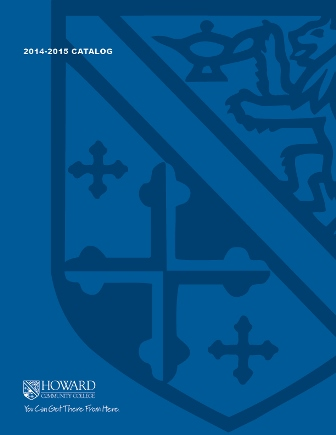2017-2018 College Catalog# MATH 132 Topics in Mathematical Literacy

This is a college-level mathematics course, designed for college students who do not expect to need college algebra, a statistics course, or a higher-level mathematics course. Five major components are included: exponential and logarithmic functions, personal finance (including interest rates and annuities), basic logical thinking, basic principles of probability, and statistical reasoning.

3

### Prerequisite

MATH 037 or MATH 070 or higher and ENGL 086 or ENGL 096 or higher, or appropriate placement scores

3 hours weekly

### Course Objectives

1. 1. Apply mathematical processes to solve problems involving exponential and logarithmic functions.
2. 2. Use appropriate formulas, algorithms, and technology to solve problems involving taxes, savings, loans, amortization tables, investments, and credit cards.
3. 3. Use logical statements in both symbolic and English form and assess the validity of logical expressions.
4. 4. Distinguish between deductive and inductive arguments (or reasoning).
5. 5. Investigate chance processes and develop, use, and evaluate probability models.
6. 6. Analyze outcomes, draw conclusions, or make decisions related to risk, pay-off, and expected value in various probability contexts.
7. 7. Identify limitations and strengths in studies and polls including analyzing data collection methods, sources of bias, margins of error, and misuses of statistics.
8. 8. Solve problems and make decisions using complex numeric summaries from studies and polls.
9. 9. Identify and implement appropriate technologies to efficiently complete tasks that involve the solving of cross-discipline, mathematically appropriate problems and creating new works to communicate the processes used and solution.

### Course Objectives

1. Apply mathematical processes to solve problems involving exponential and logarithmic functions.

2. Use appropriate formulas, algorithms, and technology to solve problems involving taxes, savings, loans, amortization tables, investments, and credit cards.

3. Use logical statements in both symbolic and English form and assess the validity of logical expressions.

4. Distinguish between deductive and inductive arguments (or reasoning).

5. Investigate chance processes and develop, use, and evaluate probability models.

6. Analyze outcomes, draw conclusions, or make decisions related to risk, pay-off, and expected value in various probability contexts.

7. Identify limitations and strengths in studies and polls including analyzing data collection methods, sources of bias, margins of error, and misuses of statistics.

8. Solve problems and make decisions using complex numeric summaries from studies and polls.

9. Identify and implement appropriate technologies to efficiently complete tasks that involve the solving of cross-discipline, mathematically appropriate problems and creating new works to communicate the processes used and solution.